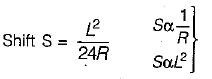Courses

# Tacheometric, Curve And Hydrographic Surveying - 1

## 10 Questions MCQ Test Topicwise Question Bank for GATE Civil Engineering | Tacheometric, Curve And Hydrographic Surveying - 1

Description
This mock test of Tacheometric, Curve And Hydrographic Surveying - 1 for Civil Engineering (CE) helps you for every Civil Engineering (CE) entrance exam. This contains 10 Multiple Choice Questions for Civil Engineering (CE) Tacheometric, Curve And Hydrographic Surveying - 1 (mcq) to study with solutions a complete question bank. The solved questions answers in this Tacheometric, Curve And Hydrographic Surveying - 1 quiz give you a good mix of easy questions and tough questions. Civil Engineering (CE) students definitely take this Tacheometric, Curve And Hydrographic Surveying - 1 exercise for a better result in the exam. You can find other Tacheometric, Curve And Hydrographic Surveying - 1 extra questions, long questions & short questions for Civil Engineering (CE) on EduRev as well by searching above.
QUESTION: 1

Solution:
QUESTION: 2

Solution:
QUESTION: 3

### The shape of the vertical curve generally provided is

Solution:
QUESTION: 4

If L is the length of transition curve and R is the radius of circular curve, then the shift of the curve is directly proportional to

Solution:QUESTION: 5

For a simple circular curve, which one of the following gives the correct relation between the radius, R and degree of curve D, for 20 m arc length?

Solution:

For 20 m arc R = 1145.9/D
for 30 m arc R = 1718.9/D

QUESTION: 6

Which of the following elements of a simple curve are correctly matched?
1. Tangent length ..... R tan Δ/2
2. Apex distance .... 2R sin Δ/2
3. Length of long chord.... 2 R cosec Δ/2
4. Mid-ordinate .... R ver sin Δ/2
(R is the radius and Δ is the deflection angle)

Select the correct answer using the codes given below:

Solution:
QUESTION: 7

If a tacheometer is fitted with an anallatic lens

Solution:
QUESTION: 8

If Δ is the angle of deflection of the curve, Tand T2 are its points of tangencies, the angle between the tangent at T1 and long chord T1 T2 will be

Solution:
QUESTION: 9

If R is the radius of the main curve, θ the angle of deflection, S the shift and L the length of the transition curve, then, total tangent length of the curve, is

Solution:
QUESTION: 10

If the intercept on a vertical staff is observed as 0.75 m from a tacheometer, the horizontal distance between tacheometer and staff station is

Solution:

Because additive constant is zero and multiplying constant is 100 for a tacheometer